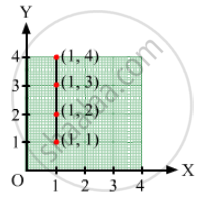Advertisement Remove all ads

# Locate the Points: (1, 1), (1, 2), (1, 3), (1, 4) - Mathematics

Answer in Brief

Locate the points:

(1, 1), (1, 2), (1, 3), (1, 4)

Advertisement Remove all ads

#### Solution

In order to plot these points, the given steps are to be followed:
Take a point O on a graph paper and draw horizontal and vertical lines OX and OY respectively.
Then, let on x-axis and y axis 1 cm represents 1 unit.
In order to plot point (1, 1), we start from the origin O and move 2 cm along OX and 1 cm along OY. The point we arrive at is (1, 1).
To plot point (1, 2), we move 1 cm along OX and 2 cm along OY. The point we arrive at is (1, 2).
To plot point (1, 3), we move 1 cm along OX and 3 cm along OY. The point we arrive at is (1, 3).
To plot point (1, 4), we move 1 cm along OX and 4 cm along OY. The point we arrive at is (1, 4).Concept: Linear Graphs
Is there an error in this question or solution?
Advertisement Remove all ads

#### APPEARS IN

RD Sharma Class 8 Maths
Chapter 27 Introduction to Graphs
Exercise 27.1 | Q 3.1 | Page 5
Advertisement Remove all ads

#### Video TutorialsVIEW ALL 

Advertisement Remove all ads
Share
Notifications

View all notifications

Forgot password?